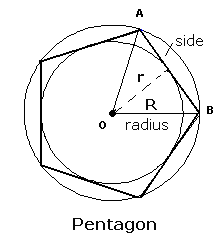### Regular polygon

A polygon is regular when all the sides and interior angles are equal, otherwise it is "irregular". The polygons are known by the number of sides. See below some examples of Regular Polygon:
1) Tetragon, 4 sided polygon;
2) Pentagon, 5 sided polygon;
3) Hexagon, 6 sided polygon;
Read full text in Regular polygon.
Enter data using the point as a decimal separator. Ex 2,230.32 enter: 2230.32; The results will be shown after a click on CalculateRegular Polygon Decimal places: Side Lenght       Excircle radius Incircle radius Number of sides Perimeter: Circumscribed circle: Area: Number of diagonals: Incircle radius polygon: Excircle radius polygon: Interior angle: Exterior angle: Sum of interior angles: Sum of exterior angles: Note:

Note:The accuracy of the calculator and its applicability to particular cases is not guaranteed.